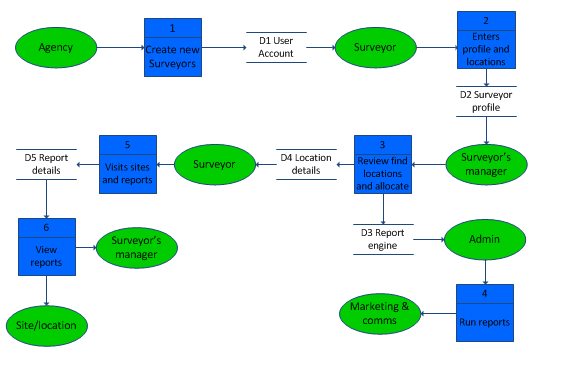# Data Flow Diagram

[Level 1 Data Flow Diagram -] this highlights the main functions carried out by the system. As a rule, we try to describe the system using between two and seven functions. SQA

## What is a data flow diagram (DFD)?

Data flow diagrams or DFDs help the analysis of systems by showing:
• Data sources
• What processing happens and by whom
• Who uses the data
• Where data is stored and when
• Outputs produced
Representing the complete business system often requires multiple levels of diagram.

The top level, is referred to Level 0 or the Context Diagram, it represents the system as one process box. Level 1 data flow diagrams show incoming data flow, processes and output data flows.
Level 1 DFDs should show a process to handle each incoming data flow and a process to generate each output data flow.

## Example Data Flow Diagrams

This example of a level 1 data flow diagrams is free to copy, download and use.## Other example data flow diagrams